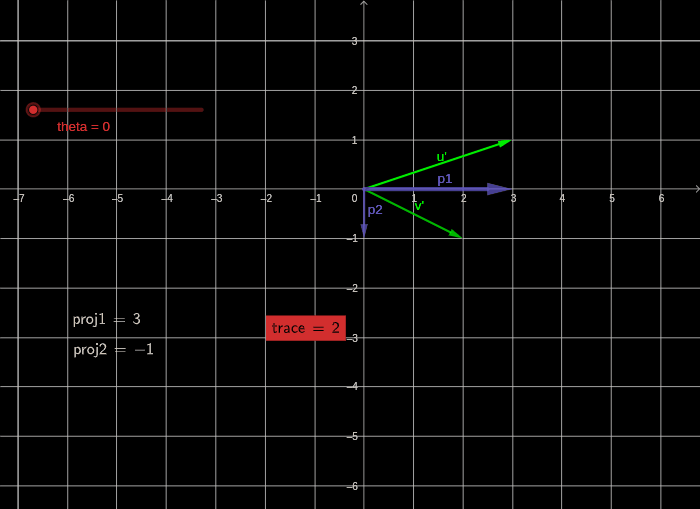# Geometric meaning of a trace

In linear algebra, the determinant of a matrix is very nicely linked to areas and volumes. For example, the determinant of a 3x3 matrix is the volume of the parallelopiped enclosed by the three columns of the matrix represented as vectors in 3D- space. To see why this is true see this video from 3blue1brown.

The volume here is 2 x 3 x 1 = 6 which is also the determinant of the matrix.

# Trace

The trace of a matrix is defined as the sum of the diagonal elements of a matrix. When I wanted to find the geometric representation of a trace I could not find one, so I decided to create one.

In this post, we will see the trace represented geometrically and then also confirm if the trace remains unchanged under a change of basis operation.
It can be proved that the change of basis (more on what is change of basis later) does not change the trace. We will try to confirm that using the geometric representation.

Consider the 2x2 matrix here.

Let’s define trace as the sum of signed projections of the columns of the matrix on the corresponding basis vectors of the space.

In this example, if we assume the standard basis to be the basis i.e. (1,0) and (0,1). Then the projections are given by:

Signed projection of (3,1) on basis vector (1,0) = 3
Signed projection of (2,-1) on basis vector (0,1) = -1

Trace = 3 + (-1) = 2

Now let’s see if the trace remains unchanged by a change of basis operation. A change of basis operation finds the new representation of the matrix in a new basis. These operations are essential when transforming from standard basis to other basis (example- eigen basis). A change of basis operation transforms the entire grid into a new grid (with the origin remaining intact) and no grid line curving.

Let’s consider a new basis — ‘rotate counterclockwise by the theta’ basis
This basis rotates everything in our standard basis by an arbitrary angle theta.

We would first want to know to a person named ThetaBoy sitting in this matrix what would our matrix T look like. For this purpose we employ the change of basis transformation. The matrix T in ThetaBoy’s basis looks like this to him

Working this out gives this matrix in the new basis. This is how the matrix will look like to ThetaBoy sitting in his basis.

Now let’s see this geometrically.

So to ThetaBoy sitting in the “rotate counterclockwise by theta basis” the matrix transformation T looks like the one shown above. Now to check if the geometric definition of a trace still adds up in this new space, we will project these matrix columns onto the (0,1) and (1,0) basis vectors. Note that these basis vectors are basis vectors for ThetaBoy in this new basis from his perspective. Now trace becomes-

Signed projection of u’ on ThetaBoy’s basis vector (1,0) = 2.5
Signed projection of v’ on ThetaBoy’s basis vector (0,1) = -0.5

Trace = 2.5–0.5= 2

Hence the trace remains same. In fact for any ‘rotate by counterclockwise theta’ basis with any theta the signed projections always add up to 2 for ThetaBoy’s sitting in the new basis’ space.Projections of matrix columns for our transformation in different basis according to theta

Therefore Trace of a matrix remains same independent of the basis in which the matrix is represented in. The geometrical representation provides us an understanding of why this might be.
The next step would be to think of the geometric representation in 3-D space and higher dimension which logically follows.

Data Engineer in a computational biology company

## More from Saksham Malhotra

Data Engineer in a computational biology company